# Functions¶

If a task has to be performed in a program many times, it is better to code that task as a function. Function is a piece of reusable code that can be invoked(called) from anywhere. They perform the intended task with supplied parameters and return the result if needed.

Python function has several advanatages over C functions and Java methods:

• Functions can take variable number of arguments. This is supported natively
• Functions can have named arguments (you had seen it in print())
• Functions can return multiple values
• If you need a helper function for a function, you can define it inside the function

## Defining a function¶

The syntax for defining a function is as follows

def function_name(argument_list):
statement_1
statement_2
...
statement_n
return values


Let’s write a function for calculating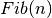,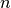‘th Fibonacci Number, defined by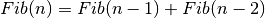, whereand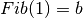First implementation uses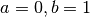. Further implementations include options for modifying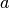and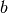In :

def fibonacci_first(n):
first,second = 0,1
while n != 0:
n, first, second = n - 1, second, first + second
return first

In :

fibonacci_first(10)       # Function call

Out:

55


Let’s have an option to chooseandIn :

def fibonacci_second(n,a,b):
first,second = a,b
while n != 0:
n, first, second = n - 1, second, first + second
return first

In :

fibonacci_second(9,1,1)

Out:

55


Letandhave the default values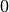andrespectively

In :

def fibonacci_third(n,a=0,b=1):
first,second = a,b
while n != 0:
n, first, second = n - 1, second, first + second
return first

In :

fibonacci_third(10)  # behaves like fibonacci_first()

Out:

55

In :

fibonacci_third(9,1) # behaves like fibonacci_second(9,1,1)

Out:

55

In :

fibonacci_third(9,1,2) # Run with fully different parameters

Out:

89


You can also change one default value. You can do this by passing named argument to function

In :

fibonacci_third(9,b=3)

Out:

102


### What we have to do if we wantFibonacci Numbers instead ofth Fibonacci Number?¶

• One soulution is to return a list ofnumbers. We will see that once we learn about Lists in next chapter
• What we can do now is return an iterable object, that iterates throughFibonacci numbers. Instead of returning a number, we can simply yield it to construct a genertor. The resulting Generator object can be used with for loop. (remember range object)
In :

def fibonacci_generator(n,a=0,b=1):
first,second = a,b
while n != 0:
yield first
n, first, second = n - 1, second, first + second

In :

for num in fibonacci_generator(10):
print(num,end=',')

0,1,1,2,3,5,8,13,21,34,


Now you can also use in operator to check the membership of an element in the Generator Object

In :

8 in fibonacci_generator(10)

Out:

True

In :

10 in fibonacci_generator(10)

Out:

False


Let’s modify above loop in order to print Fibonacci Numbers with numbering

In :

for i,num in enumerate(fibonacci_generator(10,a = 2, b = 3)):
print('Fib({})={}'.format(i,num))

Fib(0)=2
Fib(1)=3
Fib(2)=5
Fib(3)=8
Fib(4)=13
Fib(5)=21
Fib(6)=34
Fib(7)=55
Fib(8)=89
Fib(9)=144


enumerate() function takes an iterable object as an argument and returns an iterator which is the original iterator enumerated.